Courses

# Ideal Bose & Fermi Gases (Part-2) - Thermodynamic & Statistical Physics, CSIR-NET Physical Sciences Physics Notes | EduRev

## Physics for IIT JAM, UGC - NET, CSIR NET

Created by: Akhilesh Thakur

## Physics : Ideal Bose & Fermi Gases (Part-2) - Thermodynamic & Statistical Physics, CSIR-NET Physical Sciences Physics Notes | EduRev

The document Ideal Bose & Fermi Gases (Part-2) - Thermodynamic & Statistical Physics, CSIR-NET Physical Sciences Physics Notes | EduRev is a part of the Physics Course Physics for IIT JAM, UGC - NET, CSIR NET.
All you need of Physics at this link: Physics

Fermi and Bose gases

Like we did at the beginning  we consider all particles at the same quantum state as Gibbs subsystem and apply the grand canonical distribution with the potential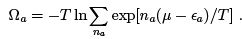(51)

Here the sum is over all possible occupation numbers na. For fermions, there are only two terms in the sum with n= 0; 1 so that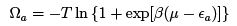For bosons, one must sum the infinite geometric progression (which converges when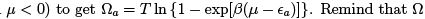depends on T ; V ; μ. The average number of particles in the state with the energy ε is thus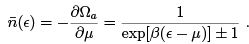(52)

Upper sign here and in the subsequent formulas corresponds to the Fermi statistics, lower to Bose. Note that at exp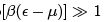both distributions turn into Boltzmann distribution (39). The thermodynamic potential of the whole system is obtained by summing over the states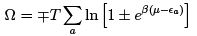(53)
Fermi and Bose distributions are generally applied to elementary particles (electrons, nucleons or photons) or quasiparticles (phonons) since atomic and molecular gases are described by the Boltzmann distribution (with the recent exception of ultra-cold atoms in optical traps). For elementary particle, the energy is kinetic energy, ε = p2/2m, which is always quasi-classical (that is the thermal wavelength is always smaller than the size of the box but can now be comparable to the distance between particles). In this case we may pass from summation to the integration over the phase space with the only addition that particles are also distinguished by the direction of the spin s so there are g = 2s + 1 particles in the elementary sell of the phase space.
We thus replace (52) by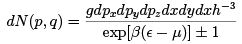(54)

Integrating over volume we get the quantum analog of the Maxwell distribution: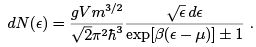(55)
In the same way we rewrite (53):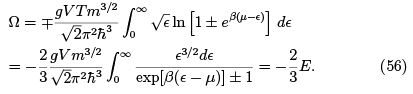Since also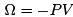we get the equation of state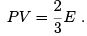(57)
We see that this relation is the same as for a classical gas, it actually is true for any non-interacting particles with ε = p2/2m in 3-dimensional space. Indeed, consider a cube with the side ℓ. Every particle hits a wall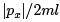times per unit time transferring the momentum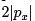in every hit. The pressure is the total momentum transferred per unit time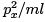divided by the wall area ℓ2 :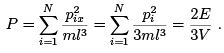(58)

In the limit of Boltzmann statistics we have E = 3N T /2 so that (57) reproduces P V = N T . Let us obtain the (small) quantum corrections to the pressure assuming exp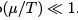Expanding integral in (56)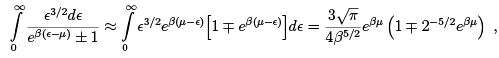and substituting Boltzmann expression for μ we get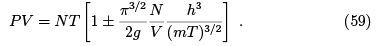Non-surprisingly, the small factor here is the ratio of the thermal wavelength to the distance between particles. We see that quantum e®ects give some e®ective attraction between bosons and repulsion between fermions.

Degenerate Fermi Gas

The main goal of the theory here is to describe the electrons in the metals (it is also applied to the Thomas-Fermi model of electrons in large atoms, to protons and neutrons in large nucleus, to electrons in white dwarf stars, to neutron stars and early Universe). Drude and Lorents at the beginning of 20th century applied Boltzmann distribution and obtained decent results for conductivity but disastrous discrepancy for the specific heat (which they expected to be 3/2 per electron). That was cleared out by Sommerfeld in 1928 with the help of Fermi-Dirac distribution. Since the energy of an electron in a metal is comparable to Rydberg and so is the chemical potential (see below) then for most temperatures we may assume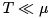so that the Fermi distribution is close to the step function: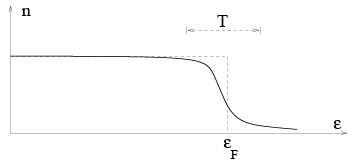At T = 0 electrons fill all the momenta up to pF that can be expressed via the concentration (g = 2 for s = 1=2):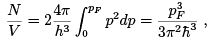(60)
which gives the Fermi energy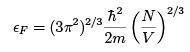(61)
The chemical potential at T = 0 coincides with the Fermi energy (putting already one electron per unit cell one obtains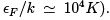Condition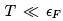is evidently opposite to (40). Note that the condition of ideality requires that the electrostatic energy Ze2/a is much less than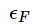where Ze is the charge of ion and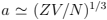is the mean distance between electrons and ions. We see that the condition of ideality,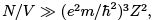surprisingly improves with increasing concentration. Note nevertheless that in most metals the interaction is substantial, why one can still use Fermi distribution (only introducing an effective electron mass) is the subject of Landau theory of Fermi liquids to be described in the course of condensed matter physics (in a nutshell, it is because the main effect of interaction is reduced to some mean effective periodic field).

To obtain the specific heat,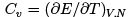one must find  E(T,V,N) i.e exclude μ from two relations, (55) and (56).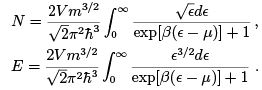At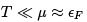this can be done perturbatively using the formula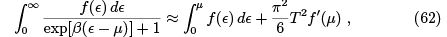which gives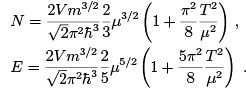From the first equation we find μ(N, T) perturbatively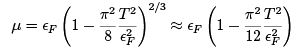and substitute it into the second equation: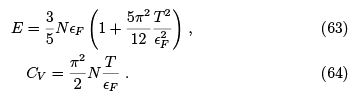we see that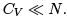Photons

Consider electromagnetic radiation in an empty cavity kept at the temperature T. Since electromagnetic waves are linear (i.e. they do not interact) thermalization of radiation comes from interaction with walls (absorption and re-emission). One can derive the equation of state without all the formalism of the partition function. Indeed, consider the plane electromagnetic wave with the fields having amplitudes E and B. The average energy density is (E2 +B2)/2 = Ewhile the momentum flux modulus is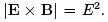The radiation field in the box can be considered as incoherent superposition of plane wave propagating in all directions. Since all waves contribute the energy density and only one-third of the waves contribute the radiation pressure on any wall then

PV =E/3

In a quantum consideration we treat electromagnetic waves as photons which are massless particles with the spin 1 which can have only two independent orientations  correspond to two independent polarizations of a classical electromagnetic wave). The energy is related to the momentum by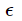= cp. Now, exactly as we did for particles [where the law= p2/2m  gave PV = 2E/3 — see (58)] we can derive (65) considering  that every incident photon brings momentum 2p cos θ to the wall, that the normal velocity is c cos θ and integrating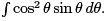Photon pressure is relevant inside the stars, particularly inside the Sun.

Let us now apply the Bose distribution to the system of photons in a cavity. Since the number of photons is not fixed then minimumality of the free energy, F(T, V, N), requires zero chemical potential: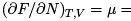0. The Bose distribution over the quantum states with fixed polarization, momentum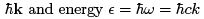is called Planck distribution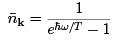At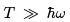it gives the Rayleigh-Jeans distribution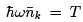which is classical equipartition. Assuming cavity large we consider the distribution over wave vectors continuous. Multiplying by 2 (the number of polarizations) we get the spectral distribution of energy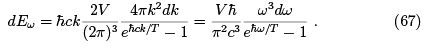It has a maximum at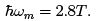The total energy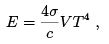(68)

where the Stephan-Boltzmann constant is as follows: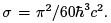The specific heat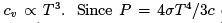depends only on temperature, cP does not exist (may be considered infinite). One can also derive the free energy (which coincides with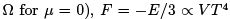and entropy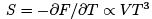that is the Nernst law is satisfied: S→0 when T → 0. Under adiabatic compression or expansion of radiation entropy constancy requires V T3 = const and PV4/3 = const.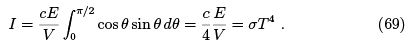Phonons

The specific heat of a crystal lattice can be calculated considering the oscillations of the atoms as acoustic waves with three branches (two transversal and one longitudinal)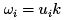where ui  is the respective sound velocity. Debye took this expression for the spectrum and imposed a maximal frequency ωmax so that the total number of degrees of freedom is equal to 3 times the number of atoms: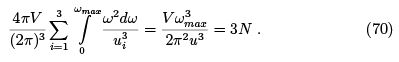Here we introduced some effective sound velocity u defined by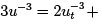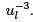One usually introduces the Debye temperature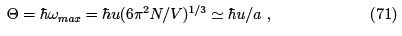where a is the lattice constant.

We can now write the energy of lattice vibrations using the Planck distribution (since the number of phonons is indefinite, μ = 0)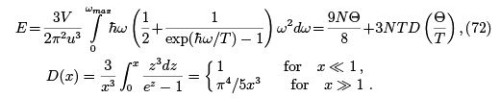At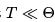for the specific heat we have the same cubic law as for photons: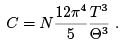(73)

For liquids, there is only one (longitudinal) branch of phonons so C =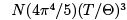which works well for He IV at low temperatures.

At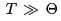we have classical specific heat (Dulong-Petit law) C = 3N. Debye temperatures of different solids are between 100 and 1000 degrees Kelvin. We can also write the free energy of the phonons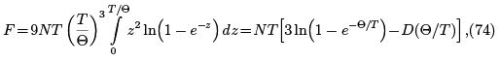and find that, again, al low temperatures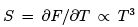Nernst theorem. An interesting quantity is the coefficient of thermal expansion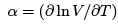To get it one must pass to the variables P, T, μ introducing the Gibbs potential G(P, T) = E − T S + PV and replacing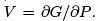At high temperatures,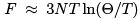It is the Debye temperature here which depends on P, so that the part depending on T and P in both potentials is linearly proportional to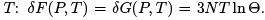That makes the mixed derivative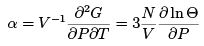independent of temperature. One can also express it via so-called mean geometric frequency defined as follows: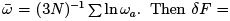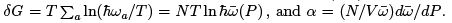When the pressure increases, the atoms are getting closer, restoring force increases and
so does the frequency of oscillations so that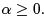Note that we’ve got a constant contribution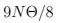in (72) which is due to quantum zero oscillations. While it does not contribute the specific heat, it manifests itself in X-ray scattering, M ̈ossbauer effect etc. Incidentally, this is not the whole energy of a body at zero temperature, this is only the energy of excitations due to atoms shifting from their equilibrium positions. There is also a negative energy of attraction when the atoms are precisely in their equilibrium position. The total (so-called binding) energy is negative for crystal to exists at T = 0.
One may ask why we didn’t account for zero oscillations when considered photons in (67,68). Since the frequency of photons is not restricted from above, the respective contribution seems to be infinite. How to make sense out of such infinities is considered in quantum electrodynamics; note that the zero oscillations of the electromagnetic field are real and manifest them-selves, for example, in the Lamb shift of the levels of a hydrogen atom. In thermodynamics, zero oscillations of photons are of no importance.

Bose gas of particles and Bose-Einstein condensation

We consider now an ideal Bose gas of massive particles with the fixed number of particles. This is applied to atoms at very low temperatures. As usual, equaling the total number of particles to the sum of Bose distribution over all states gives the equation that determines the chemical potential as a function of temperature and the specific volume. It is more convenient here to work with the function z= exp(μ/T) which is called fugacity: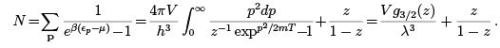We introduced the thermal wavelength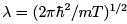and the function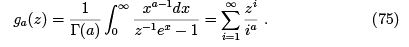One may wonder why we split the contribution of zero-energy level as it is not supposed to contribute at the thermodynamic limit V → ∞. Yet this is not true at sufficiently low temperatures. Indeed, let us rewrite it denoting n0 = z/(1-z) the number of particles at p =0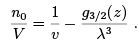(76)

The function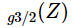behaves as shown at the figure, it monotonically grows while z changes from zero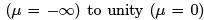. Remind that the chemical potential of bosons is non-positive (otherwise one would have infinite occupation numbers).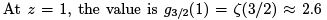and the derivative is infinite. When the temperature and the specific volume v= V/N  are such that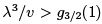(notice that the thermal wavelength is now larger than the inter-particle distance) then there is a finite fraction of particles that occupies the zero-energy level. The solution of (76) looks as shown in the figure. When V → ∞ we have a sharp transition at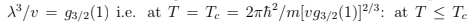we have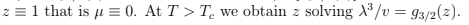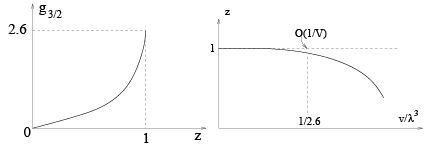Therefore, at the thermodynamic limit we put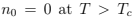and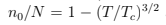as it follows from (76). All thermodynamic relations have now different expressions above and below Tc (upper and lower cases respectively):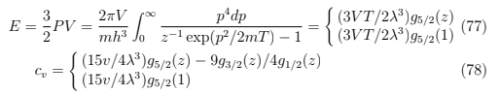At low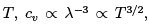it decreases faster than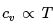for electrons (since the number of over-condensate particles now changes with T as for

phonons and photons and μ = 0 too) yet slower than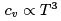(that we had  for εp= p2/2m, are denser at lower energies. On the other hand, since the distance between levels increases with energy so that at high temperatures cv decreases with T as for rotators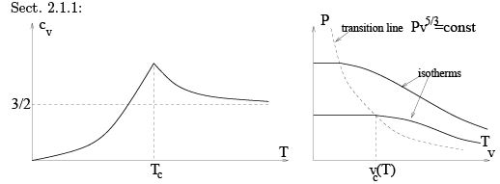At T < Tc the pressure is independent of the volume which promts the analogy with a phase transition of the first order. Indeed, this reminds the properties of the saturated vapor (particles with nonzero energy) in contact with the liquid (particles with zero energy): changing volume at fixed tem- perature we change the fraction of the particles in the liquid but not the pressure. This is why the phenomenon is called the Bose-Einstein condensation. Increasing temperature we cause evaporation (particle leaving condensate in our case) which increases cv; after all liquid evaporates (at T = Tc) cv starts to decrease. It is sometimes said that it is a “condensation in the momentum space” but if we put the system in a gravity field then there will be a spatial separation of two phases just like in a gas-liquid condensation (liquid at the bottom).

We can also obtain the entropy [above Tc by usual formulas that follow from (56) and below Tc just integrating specific heat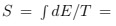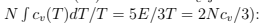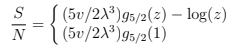(79)

The entropy is zero at T = 0 which means that the condensed phase has no entropy. At finite T all the entropy is due to gas phase. Below Tc we can write S/N = (T/Tc)3/2 s= (v/vc) s where s is the entropy per gas particle: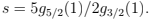The latent heat of condensation per particle is T s that it is indeed phase transition of the first order.

159 docs

,

,

,

,

,

,

,

,

,

,

,

,

,

,

,

,

,

,

,

,

,

,

,

,

;